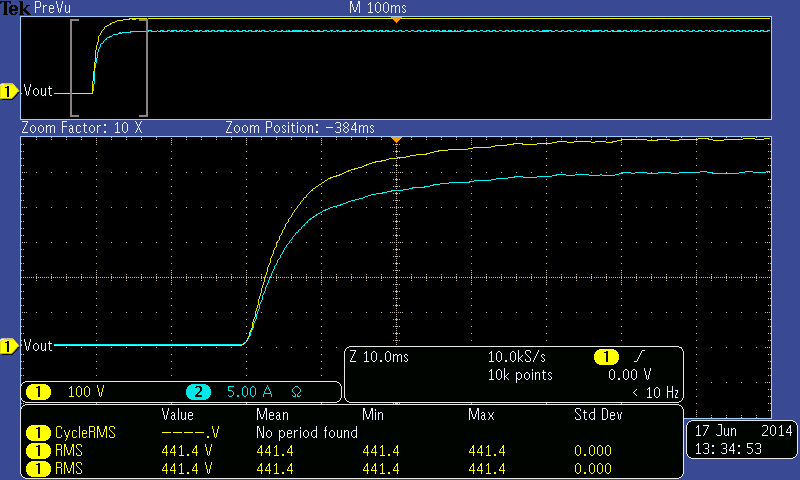# Slew rate and the available configurations

Slew rate defines the maximum rate of change per unit time for either output voltage or output current. Magna-Power's limits on slew rate produce a non-linear rise time effect. Magna-Power's slew rate specifications characterize the power supply's rise-time in response to a programmed voltage or current change. Slew rate is distinct from the power supply's much faster transient response specification, which characterizes the power supply's response to a step load change.

Magna-Power offers two configurations of its MagnaDC power supplies: standard output and High Slew Rate (+HS) output. The standard output stage MagnaDC power supplies has been designed to provide the lowest possible output ripple voltage within the constraints of available components, size, and cost. Part of the output stage consists of a bank of aluminum electrolytic capacitors which has the desired electrical properties to provide this function. While the presence of these components and the resulting performance are normally industry accepted, there are applications where lower output capacitance with faster rise and fall times are extremely desirable and higher ripple voltage is acceptable. To meet this need, a High Slew Rate (+HS) option is available which has an output stage consisting of low capacitance film and aluminum electrolytic capacitors. The High Slew Rate (+HS) option must be installed at the factory, preferably at time of order. Upgrades to the +HS option are available, requiring the unit to be shipped back to the factory for upgrade. Table 1 provides the available slew rate specifications for MagnaDC programmable DC power supplies, assuming a fully resisitive load.

Table 1. Available slew rates from MagnaDC programmable DC power supplies.
Standard Units Units with +HS Option
Voltage Slew Rate 100 ms 4 ms
Current Slew Rate 100 ms 8 ms

The slew rate specifications apply to any transition in voltage or current. For example, the same slew rate would apply for a programmed transition from 0 to 50% load as from 0 to 100%. The rise time for voltage and current can be model using Equation 1:

V(t)=V_0(1-e^-(t/T))
(1)

where V(t) is the voltage at a particular time, V0 is the commanded output voltage, t is time, and T is the first order time constant (Magna-Power's slew rate specification) in seconds.

To illustrate the slew rate specifications, a TSD600-24/208+HS power supply was connected to a fully resistive load and commanded to its full scale voltage and current: 600 Vdc and 24 Adc, respectively. The results of this experiment are shown in Figure 1. The blue trace is the current signal and the yellow trace is the voltage signal. The relative performance, about full scale voltage-current, will be consistent with the results shown in Figure 1 for models with the High Slew Rate (+HS) option.Figure 1. Voltage and current rise time on model with High Slew Rate (+HS) option.

The new voltage and current set points were issued to the power supply at -20 ms from the origin. To confirm that Equation 1 agrees with the experimental results, we can analyze the first major time division, which occurs 10 ms after the unit begins to ramp up voltage and current. At this first major time division, V0 is 600 Vdc, t is 0.01 s, and T is 0.004 s as this is a high slew rate unit. Equation 2 shows the evaluation of Equation 1, which evalutes to be consistent with the yellow trace at first major time division of Figure 1.

V(t)=600*(1-e^-(0.01/0.004))
(2)
V(t)=550.74 "[Vdc]"